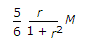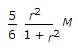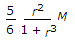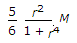# Civil Engineering - RCC Structures Design

### Exercise :: RCC Structures Design - Section 5

11.

The stresses developed in concrete and steel in reinforced concrete beam 25 cm width and 70 cm effective depth, are 62.5 kg/cm2 and 250 kg/cm2 respectively. If m = 15, the depth of its neutral axis is

 A. 20 cm B. 25 cm C. 30 cm D. 35 cm E. 40 cm

Explanation:

No answer description available for this question. Let us discuss.

12.

If the ratio of long and short spans of a two way slab with corners held down is r, the actual reduction of B.M. is given by

 A.B.C.D.E. none of these.

Explanation:

No answer description available for this question. Let us discuss.

13.

The angle of repose of a soil is the maximum angle which the outer face of the soil mass makes

 A. with the horizontal B. with the vertical C. with the perpendicular to. the inclined plane of the soil D. none of these.

Explanation:

No answer description available for this question. Let us discuss.

14.

A column is regarded as long column if the ratio of its effective length and lateral dimension, exceeds

 A. 10 B. 15 C. 20 D. 25 E. 30

Explanation:

No answer description available for this question. Let us discuss.

15.

Enlarged head of a supporting column of a flat slabs is technically .known as

 A. supporting end of the column B. top of the column C. capital D. drop panel E. none of these.